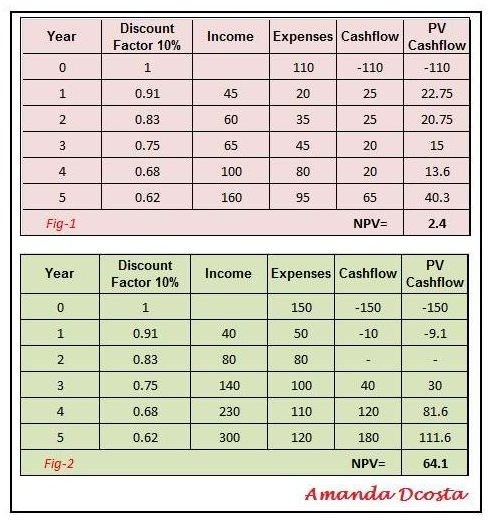# Project Management IRR - How Do You Value a Project with IRR?

Page content

## Project Management IRR

In every project, there is a debating factor of whether the project will be a success or not. Of course, since funds are used for a project, this debating factor is most often financial and determined by financial values. This brings us to the Investment Appraisal known as project management IRR (Internal Rate of Return).

Internal Rate of Return may be defined as the interest rate at which a monetary investment will return a zero Net Present Value.

Every project manager should be familiar with how IRR is used in project management, i.e., to determine the earliest time a project is able to get out of its debt phase.

## Debating a Project

Let us take an example of such a debate involving funds. A project sponsor has \$100,000 in the bank which yields him 10% interest per annum. At the end of 5 years he would have \$161,051 inclusive of the interest.

Should he decide to use these funds for a project instead; he would have to calculate the earliest time that the project breaks even for him to make a profit. If, at some point, he is able to make a profit from the project before the 5 year period of a bank deposit, the project can be rated as doable. If the project takes 5 years to break even and yield profits, then it is no better or no worse than funds deposited in the bank and serves to no purpose of investment. If the project takes more than five years to yield profits, then it would be a major disaster to invest in the project. A bank investment would be an obvious bet.

Since IRR is correlated with the Net Present Value (NPV) it is inevitable that the NPV be calculated. To calculate the NPV, we first have to calculate the Present Values of individual cash flows which gives us the current values of expected future cash flows.

Internal Rate of Return is a calculation done to nullify the effect time has over money in a project. It is a trial and error method of calculation where different interest rates are applied to the NPV. The higher the IRR, the more viable the project becomes. While NPV is expressed in terms of numerical values, IRR is expressed as percentages.

## Example Scenarios of NPV and IRRLet us take a look at how IRR is used in project management.

In the attached images, there are two examples of present value cash flow scenarios. In Figure 1, there is an initial investment of \$110 with a discount factor of 10%. Over a period of 5 years, its net present value results in 2.4. In figure 2, there is an initial investment of \$150. Over a period of 5 years, its net present value is 64.15

In these cases, if an increased discount rate is applied or higher IRR is applied, figure 1 will result in figure 3, resulting in a NPV of -9.75 with a discount factor of 14%. In the case of figure 2, if a discount factor of 19% is applied, the resulting NPV will be 0.80.

As in fig 4, when an IRR of 19% is applied to fig. 2 it results in an almost zero NPV.

Since the higher the IRR, the better the project’s feasibility, figure 4 with an IRR of 19% is a better choice from a project management point of view.